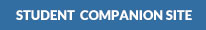Milsom, Eriksen:

Field Geophysics, 4th Edition•Figures

•Figures

•Figures

•Figures

•Figures

•Figures

•Figures

•Figures

•Figures

•Figures

•Figures

•Figures

•Figures

•Figures

•Figures

## Get Help With:

* These links will open a new window